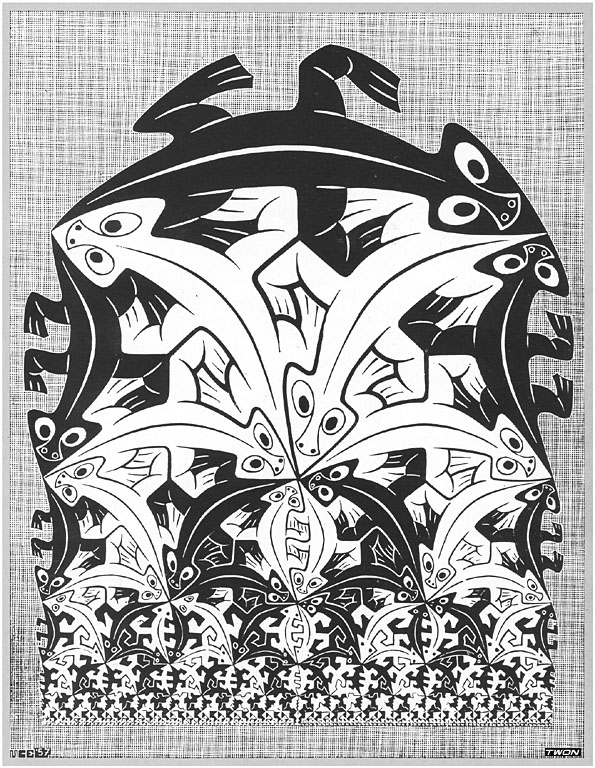# Infinite Regression: Many Pictures From One FunctionI was interested in trying to imitate Escher’s image, “Regular Division of the Plane IV”, which depicts an infinite regression of tiles towards the edge. In doing so, I realized I’d re-written a function I’d seen elsewhere- in generating Droste images- but hadn’t looked at closely to understand what it could be used for. It’s actually quite powerful and can be used to explore several of Escher’s prints! So in this post I am going to play with this function and show how you can manipulate it to generate interesting scaling tilings, as well as a square Droste image (see previous). As usual there’s an (over?)abundance of live code you can fiddle with. The code is GLSL. If GLSL is new to you, take a peek at the Book of Shaders; the editor will look familiar because I’ve borrowed it.

The equation is extremely simple:

@@f(x) = 2^{\lfloor \log_2{x}\rfloor}@@

It turns out it has the exact property we need to draw this sort of image. It’s plotted below; see also in Desmos. It builds a staircase that grows a lot like $y = x$, except that it has discrete jumps at every integer power of two. It also preserves some of the dilational symmetry of $y=x$: you can scale the whole thing by a power of two and you get the same plot. Ignoring the coloring, an extension of Escher’s print across the whole half-plane would have the same exact symmetry.

Between $0.5$ and $1.0$, $f(x) = 0.5$, and so forth, for adjacent powers of two:

@@f(x) = 2^{n} { 2^n \leq x < 2^{n+1}}@@

This is exactly what we need. If you go back and look at the Escher print, there’s a series of rows of tiles; each row is exactly half as tall as the one above, and the tiles within it are half as big.

To start with, we need lots of copies of our image. Here a circle radius $0.5$ is used to tile the plane.

We then use $f(z.x)$ as a scaling factor, and out pops the Escher-type tiling that I was interested in at the beginning! Notice how in this picture, each “column” of circles lines up with a “step” of the plot of $f$ above.

But once we’ve got this function, we can use it to draw more things! For instance, something reminiscent of Escher’s Smaller and Smaller. Instead of dividing by scale, we multiply by the reciprocal (multiplication is faster). The input to $f$ is calculated as the larger of $\left|x\right|$ and $\left|y\right|$ which produces a characteristic square pattern. The mathematics of that is either fiddly or obvious, depending on whether you’ve seen it before, so I’m going to skip over the derivation.

Looking at this, I saw the obvious similarity to Droste images; it is a Droste image, except that we’ve got extra copies of the original. “Extra copies” is a good problem to have, because we’re just a constant or two away from a classic square Droste picture.

And now we can apply Escher’s twist that I implemented previously. This time though, we don’t need to use any modulo function, since we already have our infinitely nesting copies. This makes the transformation nearly a one-liner.

The obvious generalization to $f$ is to allow an arbitrary exponent, and it turns out it also generalizes our Droste too, adjusting how deep each copy is created.

@@f(x,n) = n^{\lfloor \log_n{x}\rfloor}@@

And with some more constants and an adjustment, there’s a simple version of Escher’s Square Limit hidden inside here as well!

That’s all for now! If you want to have some more fun with Droste images, check out my previous post on fractally-distorted drostes. Or perhaps take a leap into the Time Tunnel!